Science Journal of Applied Mathematics and Statistics
Volume 4, Issue 5, October 2016, Pages: 225-228

On Application of E Linmap Model for Optimal Decision Making on Location of VIP Fast Food Restaurant in Akwa Ibom State, Nigeria

Effanga Effanga Okon, Okpara Virtue Ihuoma

Department of Statistics, Faculty of Physical & Applied Sciences, University of Calabar, Calabar, Nigeria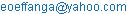(E. E. Okon)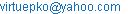(O. V. Ihuoma)

Effanga Effanga Okon, OkparaVirtue Ihuoma. On Application of E – Linmap Model for Optimal Decision Making on Location of VIP Fast Food Restaurant in Akwa Ibom State, Nigeria. Science Journal of Applied Mathematics and Statistics. Vol. 4, No. 5, 2016, pp. 225-228. doi: 10.11648/j.sjams.20160405.15

Received: August 13, 2016; Accepted: August 22, 2016; Published: September 28, 2016

Abstract: In this paper, the extended LINMAP model developed in (Effanga and Okpara, 2015) is applied to make optimal decision on location of VIP fast food restaurant in Akwa State of Nigeria. The management of the VIP fast food restaurant considered extending their services to five towns (Uyo, Eket, Ikot Ekpene, Oron and Ikot Abasi) in Akwa Ibom State. The attributes considered in the evaluation of the locations are Population, Number of retail outlets, Average family income, Start-up cost, and Taxes. The solution of our model identifies Eket as the best town to operate the business followed by Ikot Abasi, Uyo, Oron and Ikot Ekpene.

Keywords: Minkowski Metric, Optimal Decision, Utility Function, Ideal Point, Attributes

Contents

1. Introduction

Mathematical programming models have been used in a number of ways to obtain the optimal estimate of certain utility functions used in decision making (DM). Every aspect of our daily lives requires decision making which involves the use of some multiple criteria, hence, referred to as, Multi-Criteria Decision Making (MCDM) problems. Multi-criteria decision making problems are divided into Multi-Attribute (MADM) and Multi-Objective Decision Making (MODM), (Zeinab et al., 2010), (Hwang et al., 1981). In MODM the decision space is continuous, while MADM deals with problems in which set of decision alternatives have already been predetermined and decision space is discrete, (Vazifedost et al., 2011). This set of discrete decision space involves making preference decisions over a finite number of multiple attributes which are characterized by multiple and conflicting objectives, (Yoon et al., 1995). Such preference decisions making can be in evaluating, prioritizing and the selecting finite numbers of alternatives.

A MADM problem is further classified according to some defined criteria. In some cases, the decision maker is consulted for adequate information before any form of evaluation is carried out. On the other hand, the final decision is done based on some cooked data. MADM is one of the widely used decision methodology for solving most real world decision making problems, (Tuli et al., 2011). The process of selecting a method for solving MADM problems can lead to another MADM being formulated, (Triantaphyllou, 2000) and this, according to (Yeh, 2005) is caused by inconsistent ranking problem. Hence, one should be careful in selecting the method to use in handling the MADM problem since the choice of a specific method in general influences the ranking outcome, (Jahanshaloo et al., (2011).

In practice applications of some methods are not possible because of their complexities as perceived by real decision makers. Linear multi-dimensional analysis of preference (LINMAP) is one of the well-known MADM methods used (Li, 2008). It is commonly used to solve decision making problems involving multiple attributes that are conflicting in nature (Adel et al., 2012). However, in this method, the weight vector, w, and the positive ideal solution are already known. It is based on comparing the pairs of alternatives given by the decision maker and the solution with the closest distance to the positive ideal solution forms the best compromise alternative (Xia et al., 2006).

Many researchers have used or extended LINMAP to solve practical MADM problems. (Li et al., 2007) developed a fuzzy LINMAP method to handle group decision making problems involving linguistic variables with incomplete preference information to capture the uncertainty in the DM’s preferences and to calculate the distances between the alternatives and the ideal point; fuzzy goal LINMAP by (Adel et al., 2012). (Li and Yang, 2004) extended LINMAP to solve group MADM problems with fuzzy information using triangular fuzzy numbers in assessing alternatives with respect to qualitative attributes in their fuzzy linear programming model. (Effanga and Okpara, 2015) proposed an extended LINMAP (E-LINMAP) model which addresses the problem of LINMAP by providing a method of alternatives’ ranking that are devoid of ties.

The E-Linmap model (Effanga and Okpara, 2015) for the optimal estimation of utility functions of decision makers, dealt extensively with the preference of those stimuli which are closer to decision maker’s ideal point in terms of weighted Minkowski metric of order 3. This model is, hereby, applied in this paper to really buttress the point that ranking of alternatives devoid of ties can be achieved.

2. E - Linmap Model

To simultaneously determine the ideal points locations {yp} and the weights {wp} when the pre-specified locations of the ith attribute in the t-dimensional space Vi = {vip}, pϵP are given, the weighted Minkowski distance metric, di, of the ith attribute from the ideal point developed in (Effanga and Okpara, 2015) is given by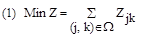Subject to: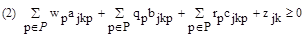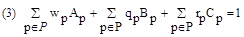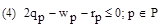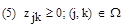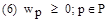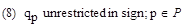Where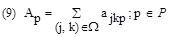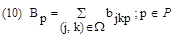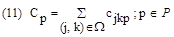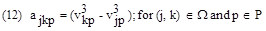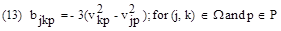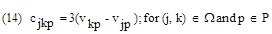3. The E - Linmap Algorithm

To estimate the weights {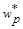} and the ideal point {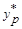}

Step 1. Let P denote the set of n attributes {1,2,...,n} and J denote the given set of m alternatives {1,2,...,m}. Let V= {vip} denote the given attribute values for the alternative (vip is the value of the ith alternative on the pth attribute). Let Ω = {(j,k)} denote the given set of ordered pairs (j,k) such that k is preferred to j on a forced choice basis in the comparison involving j and k.

Step 2. Compute ajkp and bjkp and cjkp for each pair (j,k) ϵ Ω and for every attribute pϵP. Compute Ap and Bp and Cp.

Step 3. Solve the E – LINMAP model

Step 4. Compute the index of fit C* = H*/1+H*

Step 5. Compute the distance measures and rank the alternatives.

Note that, for pϵP

(a) If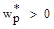then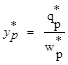(b) If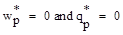define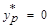(c) If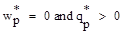then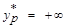(d) If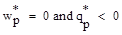then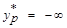4. Application

VIP fast food restaurant is growing fast in Nigeria. It has about 350 branches in Nigeria. Its management has decided to extend its services to several geographic locations in the South-South, particularly, Akwa Ibom State where it does not have any operational base. The Regional manager has identified five key towns in Akwa Ibom State where VIP does not operate or lease any fast food operations. The towns under consideration are: Oron (A1), Eket (A2), Ikot Abasi (A3), Ikot Ekpene (A4), Uyo (A5). These towns support a strong retail business base and offer varying degrees of growth in the area of population and consumer spending.

The regional manager considers using five attributes to evaluate the suitability of the five towns in Akwa Ibom State for possible extension of its services. The attributes considered are: Population (C1), Number of retail outlets (C2), Average family income (C3), Start-up cost (C4) and Taxes (C5).

The data and ratings of the towns on every attribute as given by the regional manager are given in Table 1.

Table 1. Decision Information given by the regional manager.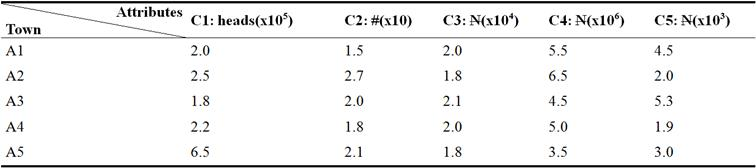The preferences between the towns (alternatives) provided by the regional manager are as follows:

Ω = {(1,2), (3,1), (4,1), (5,1), (2,3), (2,4), (2,5), (4,3), (3,5), (4,5)}

According to Table 1, the decision matrix can be obtained as follows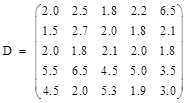Computing the parameters in our model using equations (2.1)-(3.3) we obtained the following LP model:

Min Z = Z12 + Z 31+ Z41 + Z51 + Z23 + Z24 + Z25 + Z43 + Z35 + Z45

Subject to:

Constraint 1: -4.625w1+4.058w2+2.168w3-4.816w4-265.364w5-5.2q1-3.12q2-2.28q3+4.8q4+113.52q5-1.5r1+0.6r2+0.6r3-1.2r4-13.2r5+z12 ≥ 0

Constraint 2: 9.793w2-3.42w3+2.648w4+268.793w5-9.03q2+3.51q3-2.52q4-117.03q5 +2.1r2-0.9r3+0.6r4+14.1r5+z31 ≥ 0

Constraint 3: -158.375w1-259w2-85.293w3-14.352w4+231.75w5+78.75q1+108q2

+51.03q3+60.48q4-90q5-10.5r1-12r2-8.1r3-8.4r4+9r5+z41 ≥ 0

Constraint 4: -83.125w1+7.625w2-143.045w3+3.789w4+247.625w5+48.75q1-6.75q2+74.55q3-3.69q4-99.75q5-7.5r1+1.5r2-10.5r3+0.9r4+10.5r5+z51 ≥0

Constraint 5: 4.625w1-13.851w2+1.261w3+2.168w4-3.429w5-5.25q1+12.15q2-1.23q3-2.28q4+3.51q5+1.5r1-2.7r2+0.3r3+0.6r4-0.9r5+z23 ≥ 0

Constraint 6: 163w1+254.942w2+83.125w3+119.168w4+ 33.614w5-84q1-104.88q2-48.75q3-65.28q4-23.52q5+12r1+11.4r2+7.5r3+9.6r4+4.2r5+z24 ≥ 0

Constraint 7: 87.75w1-11.68w2+140.877w3+1.027w4-+17.73w5-54q1+9.87q2-72.27q3-1.11q4-13.77q5+9r1-2.1r2+99r3+0.3r4+2.7r5+z25 ≥ 0

Constraint 8: -158.375w1-268.793w2-81.86w3-117w4-37.043w5+78.75q1

+117.03q2+47.52q3+63q4+27.03q5-10.5r1-14.1r2-7.2r3-9r4-5.1r5+z43≥0

Constraint 9: 82.125w1+2.168w2+139.616w3-1.141w4+21.168w5-48.75q1-2.28q2-71.04q3+1.17q4-17.28q5+7.5r1+0.6r2+9.6r3-0.3r4+3.6r5+z35 ≥ 0

Constraint 10: -75.25w1-266.625w2+57.725w3-118.141w4-15.875w5+30q1

+114.75q2-23.52q3+64.17q4+9.75q5-3r1-13.5r2+2.4r3-9.3r4-1.5r5+z45≥0

Constraint 11: -141.25w1-541.366w2+111.168w3-226.65w4+498.978w5+49.5q1+235.74q2-42.48q3+118.74q4-207.54q5-3r1-28.2r2+3.6r3-16.2r4+23.4r5=1

Constraint 12: w1-2q1+ r1 ≥ 0

Constraint 13: w2-2q2+ r2 ≥ 0

Constraint 14: w3-2q3+ r3 ≥ 0

Constraint 15: w4-2q4+ r4 ≥ 0

Constraint 16: w5-2q5+ r5 ≥ 0

Constraint 17: w1+w2+w3+w4+w5 = 1

w1, w2, w3, w4, w5, r1, r2, r3, r4, r5, Z12,Z31, Z41, Z51, Z23, Z24, Z25, Z43, Z35, Z45≥0

q1, q2, q3, q4, q5 unrestricted in sign

Solving the model using Microsoft Excel Solver software, the optimal solution is obtained as follows:

w1 = 0.199998

w2 = 0.199998

w3 = 0.199999

w4 = 0.200003

w5 = 0.200004

q1 = 0.106435

q2 = 0.283352

q3 = 0.509428

q4 = 0.869410

q5 = 0.447322

y1 = 0.532178

y2 = 1.416772

y3 = 2.547149

y4 = 4.347019

y5 = 2. 236585

z12 = 0.25; Z* = H* = 0.25

The Index of Fit: C* = H*/1+H* = 0.25/1+0.25 = 0.2

Therefore, the cubic distance of each town from the ideal point is calculated using Equation (15) thus

S1 = 14.32295

S2 = -2.734056

S3 = -1.976531

S4 = 52.73787

S5 = 13.86391

The ranking order of the five towns is generated as follows:

S2 < S3 < S5 < S1 < S4

The regional manager of the VIP fast food restaurant uses this ranking order to select Eket (A2) as the most attractive town in Akwa Ibom State for establishing a new branch.

5. Conclusion

The application of E-Linmap model in the choice of location of VIP Restaurant in five different towns in Akwa Ibom State yielded a remarkable result. This shows that the most preferred town is Eket. Others follow in order of preference: Ikot-Abasi, Uyo, Oron and Ikot Ekpene. Hence, the use of Minkowski metric of order 3 gives explicit result in decision making since there is more accuracy in applying it in the estimation of individual utility function in real life situation.

Acknowledgement

My appreciation goes to VIP fast food restaurant Ltd, Akwa Ibom state for their financial support during the course of this research work.

References

1. Adel, H. M.; S. Tavana; S. Saati and F. Kangi (2012). A Fuzzy Group Linear Programming Technique for Multidimensional Analysis of Preference. Journal of Intelligent and Fuzzy Systems. Vol. 1 (-1) IOSP.
2. Effanga, E. O. and Okpara, V. I. (2015):Extension of linmap model for the optimal estimation of individual utility function to minkowski distance metric of order 3. Journal Mathematical and Computing Science.
3. Hwang, C. L. and K. P. Yoon (1981) Multiple Attributes Decision Making: Methods and Applications; A State of the Art Survey. 1st Ed. Springer: Berlin Heidelberg 259pp.
4. Jahanshahloo, G. R.; M. Zohrehbandian and S. Abbasian-Naghneh (2011) Using Interactive Multiobjective Methods to Solve Multi Attribute Decision Making Problems. Australian Journal of Basic and Applied Sciences, 5(9): pp. 298-308.
5. Li, D. F. (2008). Multi-attribute group decision making methods with intuitionistic fuzzy sets. Decision and Game theory in management with intuitionistic fuzzy sets. Springer Berlin Heidelberg. 308 (2014), 251-288.
6. Li, D. F. and Yang, J. B. (2004). Fuzzy linear programming technique for multiattribute group decision making in fuzzy environments. Journal of Information Sciences, 158, 263-275.
7. Li, L., Yuan, X. H. and Z. Q. Xia (2007). Multi-criteria fuzzy decision making methods based on intuitionistic fuzzy sets. Journal of Computer and Systems sciences, 73, 84-88.
8. Triantaphyllou, E. (2000) Multi-criteria Decision making Methods: A comparative Study. London: Kluwer Academic publishers.
9. Tuli, B.; A. Sinharay and Bijan Sarkar (2011) Exploratory Analysis of Project Selection through MCDM. ICOGM-10. pp. 128-133.
10. Vazifedost, H.; M. J. Taghipouryan and M. Jarad (2011) SET for Evaluating and Ranking Banks from Viewpoint of Customers. Applied Mathematical Sciences, vol. 5, No. 11, pp. 541-556.
11. Xia, H. C., Li, D. F., Zhou, J. Y. and Wang, J. M. (2006). Fuzzy LINMAP method for multi-attribute decision making under fuzzy environments. Journal of Computer and System Sciences, 72 (2006), 741-759.
12. Yeh, C. H. (2003) The Selection of Multiattribute Decision Making Methods for Scholarship Student Selection. International Journal of Selection and Assessment, 11(4): 289-296.
13. Yoon, K. P. and C. L. Ywang (1995) Multiple Attribute Decision Making: An Introduction, Sage University Papers, 75p.
14. Zeinab, D.; M. M. Pedram and J. Shandbehzadeh (2010) A Multicriteria Decision Making Based Method For Ranking Sequential Patterns. Proceedings of the International Multiconference of Engineers and Computer Scientists. Vol. 1 IMECS 2010, Hong Kong.

 Contents 1. 2. 3. 4. 5.
Article ToolsAbstractPDF(181K)